decimals may be accomplished by changing the divisor and dividend so that the divisor becomes a whole number. Recalling that every division expression may be written in fraction form, we use the fundamental rule of fractions as follows: Rewrite the division problem as a fraction. "> Decimal DivisorsCustom SearchDecimal Divisors In the foregoing examples, the divisor in each case was an integer. Division with divisors which are decimals may be accomplished by changing the divisor and dividend so that the divisor becomes a whole number. Recalling that every division expression may be written in fraction form, we use the fundamental rule of fractions as follows: Rewrite the division problem as a fraction. Multiply the numerator (dividend) and denominator (divisor) by 10, 100, or some higher power of 10; the power of 10 must be large enough to change the divisor to a whole number. This rule is illustrated as follows:Thus 2.568 divided by 0.24 is the same as 256.8 divided by 24. From the mechanical standpoint, the foregoing rule has the effect of moving the decimal point to the right, as many places as necessary to change the divisor to an integer. Therefore the rule is sometimes stated as follows: When the divisor is a decimal, change it to a whole number by moving the decimal point to the right. Balance the change in the divisor by moving the decimal point in the dividend an equal number of places to the right. The following example illustrates this version of the rule: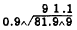The inverted v, called a caret, is used as a marker to indicate the new position of the decimal point. Notice that the decimal point in the quotient is placed immediately above the caret in the dividend. Alignment of the first quotient digit immediately above the 1 in the dividend, and the second quotient digit above the 9, assures that these digits are placed properly with respect to the decimal point. Practice problems. In the following division problems, round off each quotient to three decimal places: 1. 0.02958 ũ 0.12  2. 30.625 ũ 3.5  3. 4610 ũ 0.875 4. 0.000576 ũ 0.008 Answers: 1. 0.247  2. 8.750  3. 5268.571 4. 0.072 Dividing by Powers of 10 Division of any number by 10, 100, 1,000, etc., is really just an exercise in placing the decimal point of a decimal fraction. Thus, 5,031 + 100 may be thought of as the decimal fraction 5031/100; to remove the denominator, we simply count off two places from the right. Thus,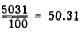The following three examples serve to illustrate this procedure further: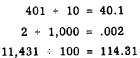If the dividend already contains a decimal part, begin counting with the first number to the left of the decimal point. Thus, 243.6 + 100 = 2.436. When the decimal point is not shown in a number, it is always considered to be to the right of the right-hand digit. Dividing by 0.1, 0.01, 0.001, etc., may also be accomplished by a simple mechanical rule. We simply begin at the position of the decimal point in the dividend and count off as many places to the right as there are decimal places in the divisor. The decimal point is then placed to the right of the last digit counted. If there are not enough digits, zeros may be added. The foregoing rule is based on the fact that 0.1 is really 1/10, 0.01 is 1/100, 0.001 is 1/1000, etc. For example,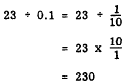Notice that dividing by 0.1 is the same as multiplying by 10. Likewise,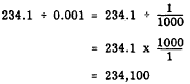andPractice problems. Divide by relocation of the decimal point. 1. 276 ũ 100 2. 2,845 ũ 1,000  3. 276 ũ 0.01 4. 2,845 ũ 0.001 Answers: 1. 2.76  2. 2.845  3. 27,600 4. 2,845,000